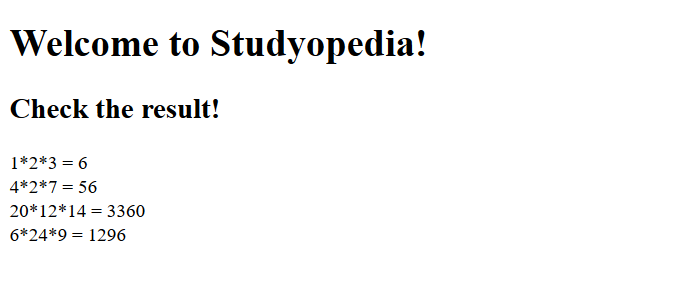# PHP Functions

## 08 Jul PHP Functions

PHP Functions are a group of statements, which takes input in the form of parameter. After taking the input, some processing is done and then the value is returned. A function, just like any other programming language, is a block of statement, which you can use repeatedly in a program. Call to the function executes it.

There are many built-in functions available, but with PHP, you can easily create a new one also. Let’s learn how to create a function in PHP,

## PHP User Defined Function

Here, we will learn how to create a function in PHP. Also, we will see how we can call that function.

To create a user defined function in PHP, use the keyword function. With PHP, you can start a function name with letter or underscore. Remember, function names in PHP are not case sensitive i.e. functionNAME is equal to functionname.

Here’s the syntax,

### Example 1

Here’s an example to create user defined function,

Here’s the output,In the above example, welcomeMsg is our user defined function and we’re printing a message using PHP echo.

### Example 2

Here’s a simple function, Display is written. Under the function, we’re printing a line.

Here’s the output,## Add parameters to PHP function

What if you need to pass information to functions? For that, you need to use parameters. These parameters are placed inside the parentheses of a function in the form of a variable.

You can add more than one parameter inside a PHP function, but separate each of them with a comma. We will learn how to add parameters to a function. Calling a function with more than one parameter is what we will see in the example below,

Let’s see the syntax for let’s say two parameters:

Let’s see some examples,

### Example 1

The following example has a function for country, with one parameter \$name. On calling this function, the country name Australia is passed, which is then displayed using PHP echo.

Here’s the output,### Example 2

Another PHP function example to showcase the usage of more than one parameters. The following example has a function for calculation, with three parameters \$x, \$y and \$z.

On calling the function, the three values are passed, which is then multiplied to display the result.

Here’s the output,### Example 3

In the below example, we have created a function, newRankings with two parameters, \$rank and \$point. We then perform calling our function, newRankings with two parameters i.e. newRankings(1, 100)

Here’s the output,## PHP Function Parameters Default Value

We will learn how to use a default value for function parameters. The default value is considered when the function having parameter is called without parameters.

Let’s see an example. Here, we’ll be printing rank of a student using PHP functions. The rank will be published as default value of function.

Here’s the output,In the above example, we have a function showRank, which is called with the default value and the result is printed.

## PHP Function return statement

For returning values from a function in PHP, use return statement. Like any other programming languages, a function in PHP can easily return a value. This is done through the return statement. More than one value can be returned with the return statement.

Let’s see some examples:

### Example 1

In the following example, we have three parameters. We will be calculating the multiplication of the three numbers and use the return statement to return the result.

Our function is displayCalc, with three parameters \$x, \$y, and \$z. The return statement returns the result in \$z as shown below:

Here’s the output,### Example 2

Here, we’re returning the result in the variable \$c. The result id the division of two numbers passed as parameters.

Here’s the output,[email protected]

We work to create free tutorials for all.